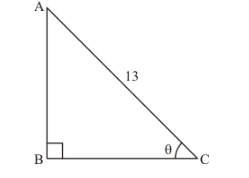# If sec θ=135, show that 2 sin θ−3 cos θ4 sin θ− 9 cos θ=3.

Question:

If $\sec \theta=\frac{13}{5}$, show that $\frac{2 \sin \theta-3 \cos \theta}{4 \sin \theta-9 \cos \theta}=3$.

Solution:

Given:

$\sec \theta=\frac{13}{5}$

To show that $\frac{2 \sin \theta-3 \cos \theta}{4 \sin \theta-9 \cos \theta}=3$

Now, we know that $\cos \theta=\frac{1}{\sec \theta}$

Therefore,

$\cos \theta=\frac{1}{\frac{13}{5}}$

Therefore,

$\cos \theta=\frac{5}{13}$...(1)

Now, we know that

$\cos \theta=\frac{\text { Base side adjacent to } \angle \theta}{\text { Hypotenuse }}$....(2)

Now, by comparing equation (1) and (2)

We get,

Base side adjacent to $\angle \theta=5$

And

Hypotenuse = 13Therefore from above figure

Base side $B C=5$

Hypotenuse $A C=13$

Side AB is unknown, It can be determined by using Pythagoras theorem

Therefore by applying Pythagoras theorem

We get,

$A C^{2}=A B^{2}+B C^{2}$

Therefore by substituting the values of known sides

We get,

$13^{2}=A B^{2}+5^{2}$

Therefore,

$A B^{2}=13^{2}-5^{2}$

$A B^{2}=169-25$

$A B^{2}=144$

$A B=\sqrt{144}$

Therefore,

$A B=12 \ldots \ldots(3)$

Now, we know that

$\sin \theta=\frac{\text { Perpendicular side opposite to } \angle \theta}{\text { Hypotenuse }}$

Now from figure (a)

We get,

$\sin \theta=\frac{A B}{A C}$

Therefore,

$\sin \theta=\frac{12}{4}$...(4)

Now L.H.S. of the equation to be proved is as follows

L.H.S. $=\frac{2 \sin \theta-3 \cos \theta}{4 \sin \theta-9 \cos \theta}$

Substituting the value of $\cos \theta$ and $\sin \theta$ from equation (1) and (4) respectively

We get,

$L . H . S .=\frac{2\left(\frac{12}{13}\right)-3\left(\frac{5}{13}\right)}{4\left(\frac{12}{13}\right)-9\left(\frac{5}{13}\right)}$

Therefore,

L.H.S. $=\frac{2 \times 12-3 \times 5}{4 \times 12-9 \times 5}$

L.H.S. $=\frac{24-15}{48-45}$

$L . H . S .=\frac{9}{3}$

$L . H . S .=3$

Hence proved that,

$\frac{2 \sin \theta-3 \cos \theta}{4 \sin \theta-9 \cos \theta}=3$